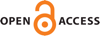#### Vol. 9, No. 5, 2016

 Download this articleFor screen For printingRecent IssuesThe Journal About the Journal Editorial Board Editors’ Interests Subscriptions Submission Guidelines Submission Form Policies for Authors Ethics Statement ISSN: 1944-4184 (e-only) ISSN: 1944-4176 (print) Author Index Coming Soon Other MSP Journals
Some nonsimple modules for centralizer algebras of the symmetric group

### Craig Dodge, Harald Ellers, Yukihide Nakada and Kelly Pohland

Vol. 9 (2016), No. 5, 877–898##### Abstract

James classified the simple modules over the group algebra $k{\Sigma }_{n}$ using modules denoted ${D}^{\lambda }$, where $\lambda$ is a partition of $n$. In particular, he showed that ${D}^{\lambda }$ is simple or zero for every partition $\lambda$ and, furthermore, that for every simple $k{\Sigma }_{n}$-module $S$ there exists a partition $\lambda$ such that ${D}^{\lambda }\cong S$. This paper is an extension of a paper of Dodge and Ellers in which they studied analogous modules ${\mathsc{D}}^{\left(\lambda ,\mu \right)}$ over the centralizer algebra $k{\Sigma }_{n}^{{\Sigma }_{l}}\phantom{\rule{0.3em}{0ex}}$, where $\lambda$ is a partition of $n$ and $\mu$ a partition of $l$. For every positive prime $p$ we find counterexamples to their conjecture that the $k{\Sigma }_{n}^{{\Sigma }_{l}}$-modules ${\mathsc{D}}^{\left(\lambda ,\mu \right)}$ are always simple or zero, where $k$ is a field of characteristic $p$. We also study the relationship between ${\mathsc{D}}^{\left(\lambda ,\mu \right)}$ and ${Hom}_{k{\Sigma }_{l}}\left({D}^{\mu },{res}_{{\Sigma }_{l}}^{{\Sigma }_{n}}{D}^{\lambda }\right)$ in special cases.

##### Keywords
centralizer algebras, symmetric groups, modular representations
##### Mathematical Subject Classification 2010
Primary: 20C05, 20C20
##### Milestones